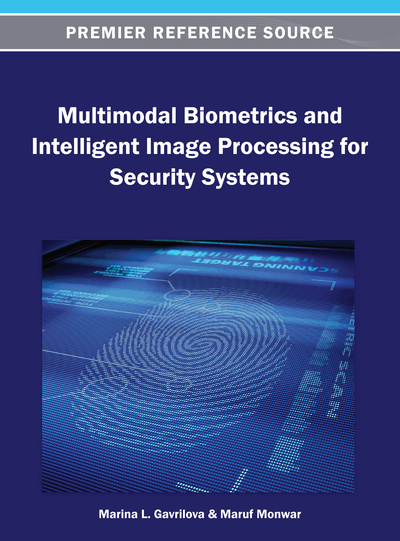# Fuzzy Fusion for Multimodal Biometric

DOI: 10.4018/978-1-4666-3646-0.ch007
Available
\$37.50
No Current Special Offers

## Abstract

Fuzzy logic is a mathematical tool that can provide a simple way to derive a conclusion with the presence of noisy input information. It is a powerful intelligent tool and used heavily in many cognitive and decision-making systems. In this chapter, fuzzy logic-based fusion approach for multimodal biometric system is discussed. After discussing the basics of fuzzy logic, the fuzzy fusion mechanism in the context of a multimodal biometric system is illustrated. A brief discussion on the research conducted for fuzzy logic-based fusion in different application domains is also presented. The biggest advantage of the system is that instead of binary “Yes”/“No” decision, the probability of a match and confidence level can be obtained. A fuzzy fusion-based biometric system can be easily adjusted by controlling weight assignment and fuzzy rules to fit changing conditions. Some results of experimentations conducted in a recent research investigation on two virtual multimodal databases are presented. The discussion on the effect of incorporating soft biometric information with the fuzzy fusion method to make the system more accurate and robust is also included.
Chapter Preview
Top

## 2. Fuzzy Logic Basics

Fuzzy logic refers to the theories and technologies that employ fuzzy sets, which are classes with un-sharp boundaries (Pedrycz & Gomide, 1998). The idea of fuzzy sets was introduced in 1965 by Professor Lotfi A. Zadeh from the University of California, Berkeley (Zadeh, 1965). The core technique of fuzzy logic is based on following four basic concepts (Wang, 2009):

• Fuzzy Sets: A fuzzy set is a set with a smooth boundary. Fuzzy set theory generalizes the classical set theory to allow partial membership (Harb & Al-Smadi, 2006).

• Linguistic Variable: A linguistic variable in one which allows its value to be described both qualitatively by a linguistic term and quantitatively by a corresponding membership function (which represents the meaning of the fuzzy set) (Harb & Al-Smadi, 2006).

• Possibility Distributions: Assigning a fuzzy set to a linguistic variable constrains the value of the variable: it generalizes the difference between possible and impossible to a degree called the possibility (Pedrycz & Gomide, 1998).

• Fuzzy Rules: Fuzzy rule (or the fuzzy if-then rule) is the most widely used technique developed using fuzzy sets and has been applied to many disciplines. Some of the applications of fuzzy rules include control (robotics, automation, tracking, consumer electronics), information systems (DBMS, information retrieval), pattern recognition (image processing, machine vision), decision support (adaptive HMI, sensor fusion), and cognitive informatics (Pedrycz & Gomide, 1998).

The development of fuzzy rule-based inference consists of three steps – fuzzification, inference and defuzzification (Figure 1) (Zadeh, 1965). In the fuzzification step, fuzzy variables and their membership functions are defined, i.e., the degree to which the input data match the condition of the fuzzy rules have been calculated. In the inference step, fuzzy rules have been developed and those rules outcome based on their matching degree has been calculated. In the defuzzification step, the fuzzy conclusion is converted into a discrete one (Zadeh, 1965).

## Complete Chapter List

Search this Book:
Reset